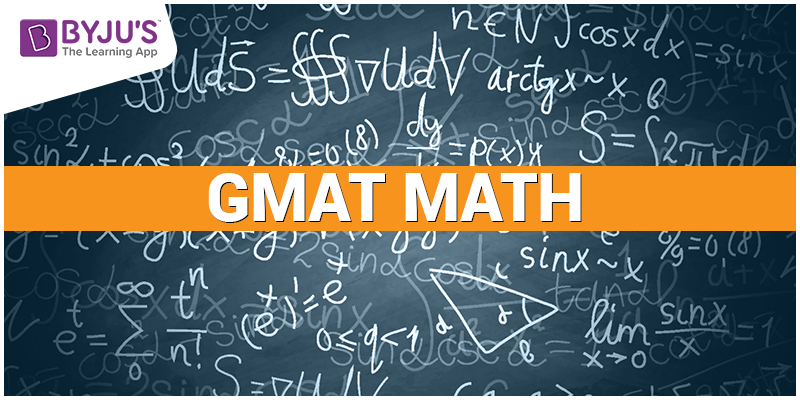# GMAT Math: Practice Test$1. \; If \; q\neq 3 \; and \; 3\frac{p}{q} \; is \; a \; number \; greater \; than \; 2, \; which \; of \; the \; following \; must \; be \; true.$ $a) \; p = q; \; b) \; q = (-1); \; c) \; p \; and \; q \; are \; prime \; integers$
1. None
2. a only
3. b only
4. c only
5. a and c
$2. If \; x \; and \; y \; are \; positive \; integer \; and \; x \; is \; a \; prime \; factor \; of y^{2}, \; which \; of \; the \; following \; must \; be \; true?$
1. x is even
2. x =  y2
3. x/y 1
4. y/x is even
5. X+1 is also a prime factor of  y2

3. A and B cycle on a circular track. A completes one round after 60 minutes and B completes one round after every 30 minutes. Provided they start together, when will they meet for the third time?

1. 60 min
2. 180 min
3. 120 min
4. 90 min
5. 40 min

4. Out of seven models, all of different heights, five models will be chosen to pose for a photograph. If five models are to stand in a line from shortest to tallest, and the fourth tallest and the sixth tallest model cannot be adjacent, How many arrangements are possible if the fourth tallest and the sixth tallest model are always chosen?

1. 6
2. 11
3. 17
4. 72
5. 210

5. How many positive integers less than 10000 are such that the product of their digit is 210?

1. 24
2. 30
3. 48
4. 54
5. 72

6. If the line P defined by the equation 2y – 10x =5 and line Q is defined by the equation 10y +2x +40 = 0. If line R does not interest line P. What is the degree measure of the line of the angle formed by the line R and line Q?

1. 0
2. 30
3. 90
4. 45
5. Cannot be determined

7. ABDE is a trapezium having area as 9. C is a point on DE such that ABCD and ABEC are parallelograms. WHat the ratio of the area of ABCD and ABEC?

1. 2
2. 4
3. 3
4. 1.5
5. 1

8. In a rabbit family, for each of the first months of life, the probability that a rabbit will die during that month is 0.3. For agoup of 200 newborn rabbits of the entire population, approximately how many would be expected to survive the first 3 months of life?

1. 10
2. 5
3. 20
4. 40
5. 60

9. An ant can move along a 6 x 4 grid taking 1 step at a time either in +ve y direction or +ve x direction. What is  the probability that it will pass through (2,3)

1. 5/21
2. 3/20
3. 1/21
4. 4/21
5. 1/10

10. Of the film released last year, 60% were comedies and the rst were horro films. 75% of the comedies were profitable. But 75% of the horror movies were unprofitable. If the studio made a total of 40 films, and broke even on none of them, how many of their films were profitable?

1. 18
2. 19
3. 20
4. 21
5. 22

1. B
2. C
3. B
4. C
5. D
6. C
7. E
8. B
9. D
10. E